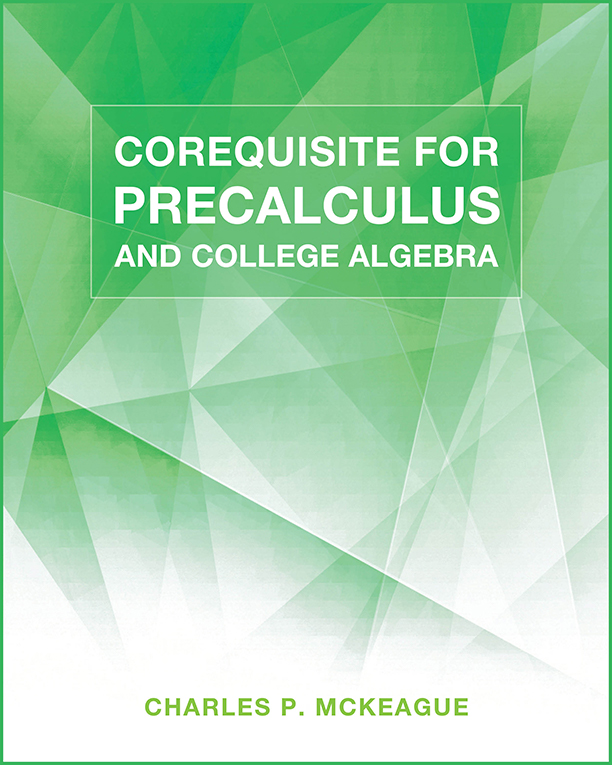## Corequisite for College Algebra and Precalculus

### Author: Charles P. McKeagueThe print book is free when you purchase any book or pass from our site.

Lessons:

Lesson 1: Definitions, Properties, and Simplifying Expressions
Lesson 2: Exponents and Scientific Notation
Lesson 3: Polynomials: Sums, Differences, and Products
Lesson 4: Review of Factoring
Lesson 5: Rational Expressions
Lesson 7: Complex Numbers
Lesson 8: Linear and Quadratic Equations
Lesson 10: Additional Equations and Formulas
Lesson 11: More Applications and Modeling
Lesson 12: Linear, Quadratic, and Rational Inequalities
Lesson 13: Equations and Inequalities with Absolute Value
Lesson 14: Paired Data and Graphing
Lesson 15: Introduction to Functions and Relations
Lesson 16: Function Notation and More Graphing
Lesson 17: Transformations and Other Graphing Techniques
Lesson 18: Algebra and Composition with Functions
Lesson 19: The Slope of a Line
Lesson 20: Linear Functions and Equations of Lines